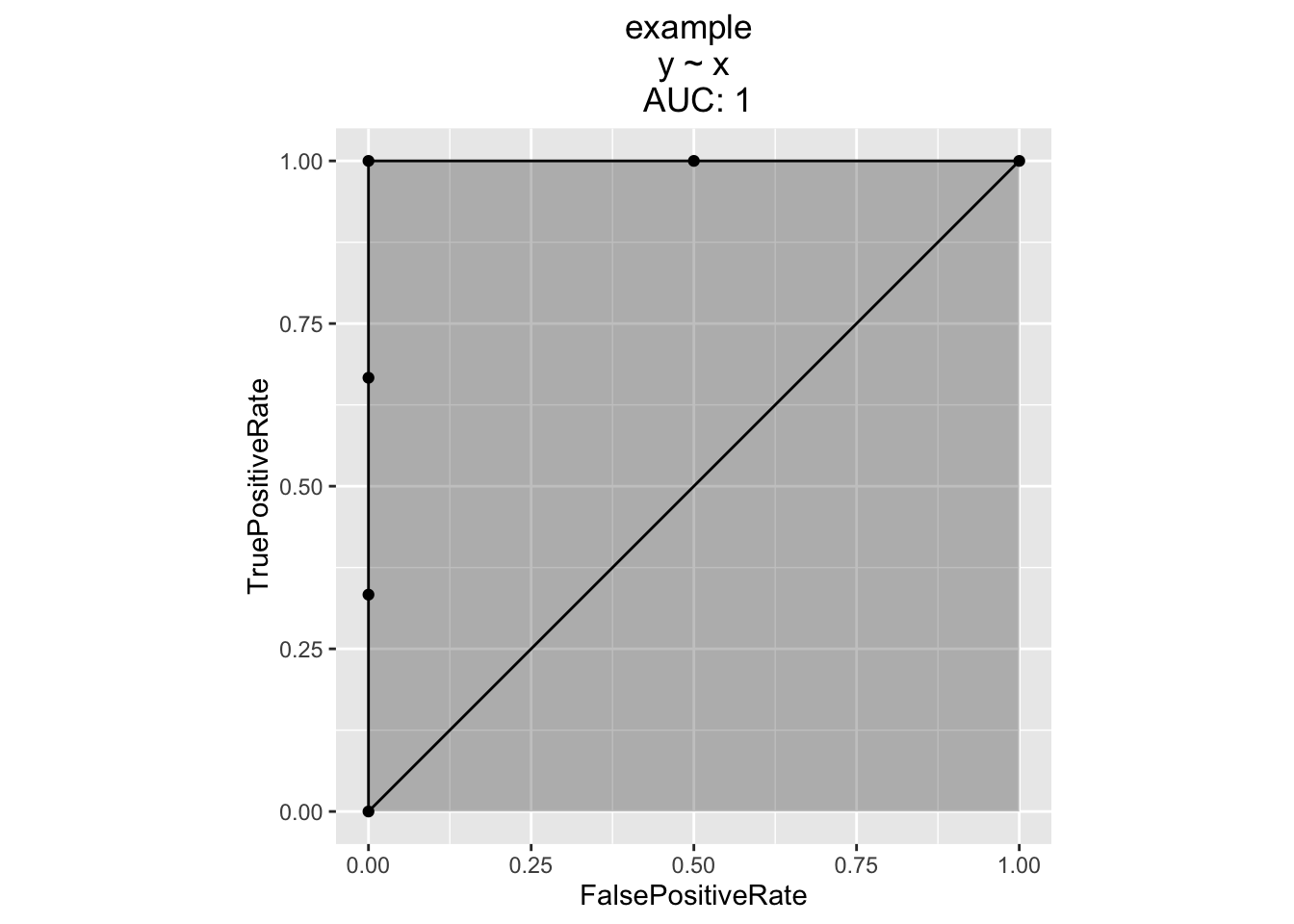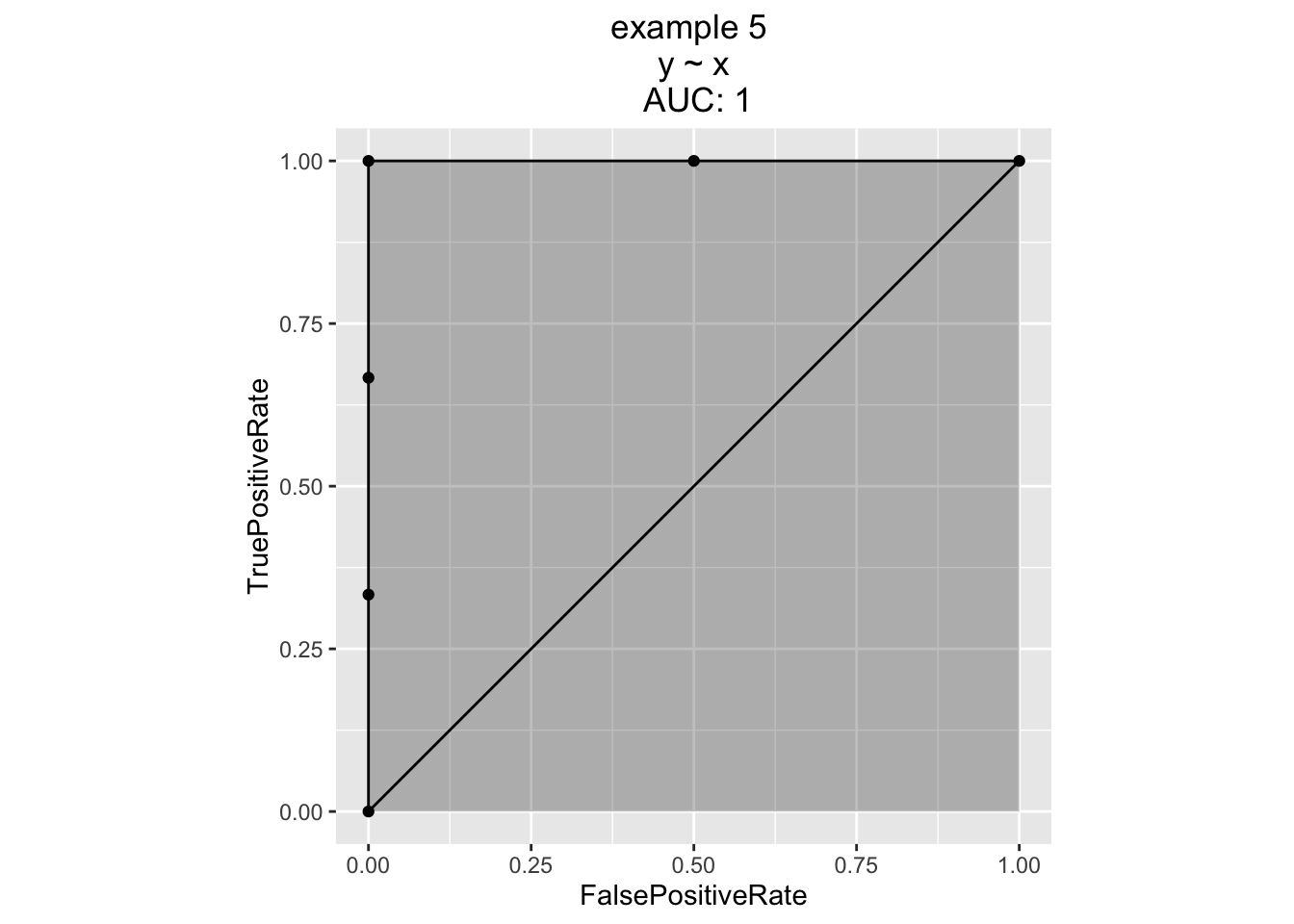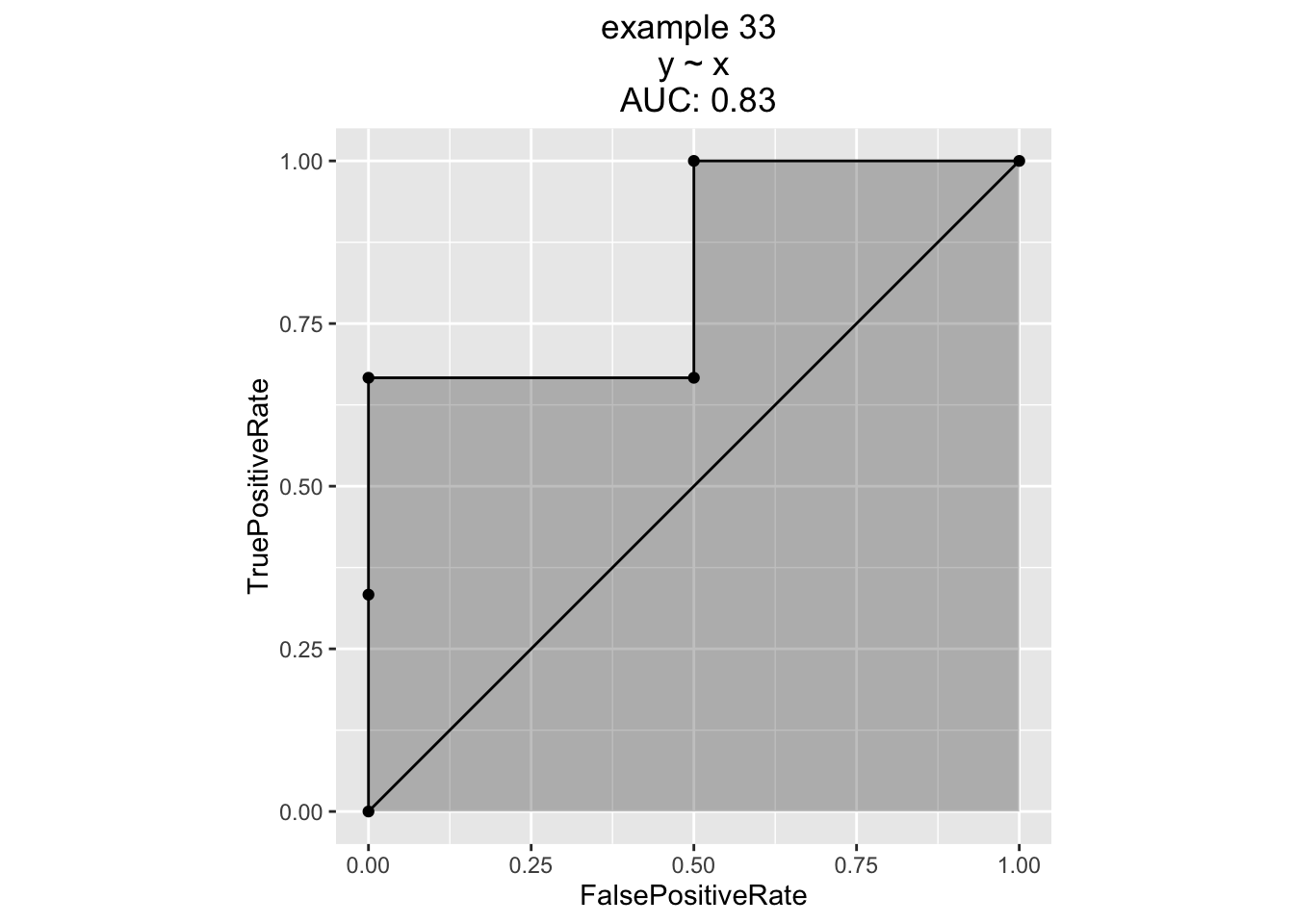## Warning: package 'ggplot2' was built under R version 3.2.4
## Loading required package: gplots
## Warning: package 'gplots' was built under R version 3.2.4
##
## Attaching package: 'gplots'
## The following object is masked from 'package:stats':
##
##     lowess
# debug runner from https://github.com/WinVector/WVPlots/blob/master/R/DebugFn.R
#' Capture arguments of exception throwing plot for later debugging.
#'
#' Run fn and print result, save arguments on failure.  Use on systems like ggplot()
#' where some calculation is delayed until print().
#'
#' @param saveFile path to save RDS to.
#' @param fn function to call
#' @param ... arguments for fn
#' @return fn(...) normally, but if f(...) throws an exception save to saveFile RDS of list r such that do.call(r$fn,r$args) repeats the call to fn with args.
#'
#' @examples
#'
#' d <- data.frame(x=1:5)
#' DebugPrintFn('problem.RDS','PlotDistCountNormal',d,xvar='x','example')
#' tryCatch(
#'    DebugPrintFn('problem.RDS','PlotDistCountNormal',
#'       d,xvar='xmisspelled','example'),
#'    error = function(e) { print(e) })
#'
#' @export
DebugPrintFn <- function(saveFile,fn,...) {
args <- list(...)
tryCatch({
res = do.call(fn,args)
print(res)
res
},
error = function(e) {
saveRDS(object=list(fn=fn,args=args),file=saveFile)
stop(paste0("Wrote '",saveFile,"' on catching '",as.character(e),"'"))
})
}
# Run once, seems okay
set.seed(25352)
d <- pretendBigExpensiveExperiment()
print(ROCPlot(d,'x','y','example'))# Run a lot of important experiment
for(runNum in seq_len(100)) {
d <- pretendBigExpensiveExperiment()
print(ROCPlot(d,'x','y',paste('example',runNum)))
}
## Error in ROCR::prediction(predcol, outcol): Number of classes is not equal to 2.
## ROCR currently supports only evaluation of binary classification tasks.# Run a lot of important experiment
# not the exact same set of experiments as we didn't reset pseudo-random seed!
for(runNum in seq_len(100)) {
d <- pretendBigExpensiveExperiment()
DebugPrintFn('problem.RDS','ROCPlot',d,'x','y',paste('example',runNum))
}
## Error in value[[3L]](cond): Wrote 'problem.RDS' on catching 'Error in ROCR::prediction(predcol, outcol): Number of classes is not equal to 2.
## ROCR currently supports only evaluation of binary classification tasks.
## 'problem <- readRDS('problem.RDS')
print(problem)
## $fn ##  "ROCPlot" ## ##$args
## $args[] ## x y ## 1 1.14402934 FALSE ## 2 -0.68807307 FALSE ## 3 0.05036758 FALSE ## 4 -0.72399384 FALSE ## 5 0.72194744 FALSE ## ##$args[]
##  "x"
##
## $args[] ##  "y" ## ##$args[]
##  "example 34"
do.call(problem$fn,problem$args)
## Error in ROCR::prediction(predcol, outcol): Number of classes is not equal to 2.
## ROCR currently supports only evaluation of binary classification tasks.